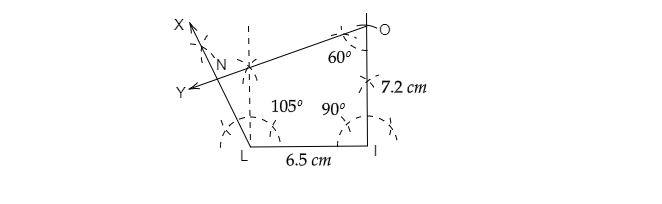# Construction a quadrilateral $LION$ with $LI=6.5\ cm$, $IO=7.2\ cm$, $\angle I=90^o$, $\angle O = 60^o$ and $\angle N=105^o$.

Given: A quadrilateral $LION$ with $LI=6.5\ cm,\ IO=7.2\ cm,\ \angle I=90^o,\ \angle O = 60^o$ and $\angle N=105^o$.

To do: To construct the quadrilateral $LION$.

Solution:As given, In quadrilateral $LION$: $LI=6.5\ cm,\ IO=7.2\ cm,\ \angle I=90^o,\ \angle O = 60^o$ and $\angle N=105^o$

$\Rightarrow \angle L +\angle I +\angle O+\angle N=360^o$

$\Rightarrow \angle L+90^o+60^o+105^o=360^o$

$\Rightarrow \angle L+255^o=360^o$

$\Rightarrow \angle L=360^o-255^o$

$\Rightarrow \angle L=105^o$

1. Draw $LI=6.5\ cm$.

2. Draw an angle $\angle XLI=105^o$ at point $L$.

3. Draw an angle $\angle I=90^o$ at point $I$.

4. Take point $I$ as center and draw an arc with radius $7.2\ cm$ which intersects the line at $O$.

5. At $O$, draw an angle $\angle YOI=60^o$.

6. $OY$ intersects $LX$ at $N$

Thus, $LION$ is the required quadrilateral.